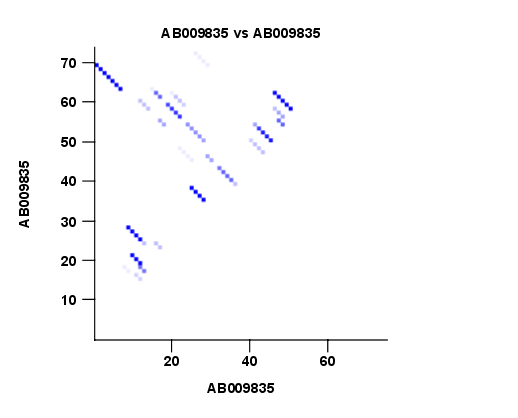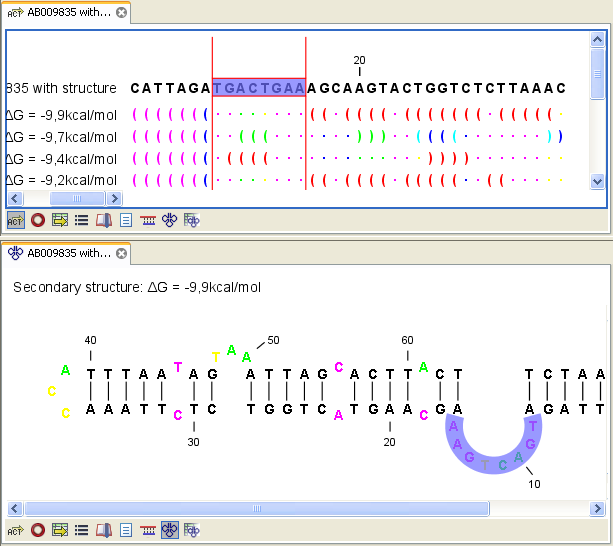## Partition function

The predicted minimum free energy structure gives a point-estimate of the structural conformation of an RNA molecule. However, this procedure implicitly assumes that the secondary structure is at equilibrium, that there is only a single accessible structure conformation, and that the parameters and model of the energy calculation are free of errors.

Obvious deviations from these assumptions make it clear that the predicted MFE structure may deviate somewhat from the actual structure assumed by the molecule. This means that rather than looking at the MFE structure it may be informative to inspect statistical properties of the structural landscape to look for general structural properties which seem to be robust to minor variations in the total free energy of the structure (see [Mathews et al., 2004]).

To this end CLC Genomics Workbench allows the user to calculate the complete secondary structure partition function using the algorithm described in [Mathews et al., 2004] which is an extension of the seminal work by [McCaskill, 1990].

There are two options regarding the partition function calculation:

• Calculate base pair probabilities. This option invokes the partition function calculation and calculates the marginal probabilities of all possible base pairs and the the marginal probability that any single base is unpaired.
• Create plot of marginal base pairing probabilities. This creates a plot of the marginal base pair probability of all possible base pairs as shown in figure 22.3.Figure 22.3: The marginal base pair probability of all possible base pairs.Figure 22.4: Marginal probability of base pairs shown in linear view (top) and marginal probability of being unpaired shown in the secondary structure 2D view (bottom).

The marginal probabilities of base pairs and of bases being unpaired are distinguished by colors which can be displayed in the normal sequence view using the Side Panel - see Structure in sequence view and also in the secondary structure view. An example is shown in figure 22.4. Furthermore, the marginal probabilities are accessible from tooltips when hovering over the relevant parts of the structure.